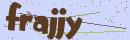PHP将大量数据导出为Excel的解决方式及性能测试# PHP将大量数据导出为Excel的解决方式及性能测试

2021-01-02 00:15:59

Windows 10

PHP 7.3.4

MySQL 8.0.12

Laravel 6.20```public function getData(\$toArray = true, \$size = 10000)
{
\$rows = \$this->limit(\$size)
->select('id', 'name', 'content', 'created_at')
->orderBy('id', 'ASC')
->get();

if (\$toArray) {
return \$rows->toArray();
}
return \$rows;
}```

`php artisan make:command PhpSpreadsheetExport`

```public function handle()
{
\$start = microtime(true);

\$this->export();

\$time = microtime(true) - \$start;

\$this->info("所需耗时{\$time}秒");
}

private function export()
{

\$modelPost = new Post();
\$rowsPost = \$modelPost->getData(true, 10000);

\$title = ['ID', '名称', '内容', '创建时间'];

foreach (\$title as \$key => \$value) {
// 单元格内容写入
\$sheet->setCellValueByColumnAndRow(\$key + 1, 1, \$value);
}

\$row = 2; // 从第二行开始 因为上面的标题已经占用了一行
foreach (\$rowsPost as \$item) {
\$column = 1;
foreach (\$item as \$value) {
// 单元格内容写入
\$sheet->setCellValueByColumnAndRow(\$column, \$row, \$value);
\$column++;
}
\$row++;
}

}```

```use PhpOffice\PhpSpreadsheet\Spreadsheet;

`php artisan excel:PhpSpreadsheet`

Laravel Excel

```<?php

namespace App\Exports;

use App\Model\Post;
use Maatwebsite\Excel\Concerns\FromCollection;

class PostExport implements FromCollection
{
public function collection()
{
\$modelPost = new Post;
return \$modelPost->getData();
}
}```

```public function handle()
{
\$start = microtime(true);

\$this->export();

\$time = microtime(true) - \$start;

\$this->info("所需耗时{\$time}秒");
}

private function export()
{
}```

php-ext-xlswriter

```public function handle()
{
\$start = microtime(true);

\$this->export();

\$time = microtime(true) - \$start;

\$this->info("所需耗时{\$time}秒");
}

private function export()
{
\$modelPost = new Post;
\$rowsPost = \$modelPost->getData(true, 10000);

// 进行数据组装 如果你有更好的方法可以不这样
\$lstPost = [];
foreach (\$rowsPost as \$rowPostKey => \$rowPost) {
\$lstPost[\$rowPostKey] = [
\$rowPost['id'],
\$rowPost['name'],
\$rowPost['content'],
\$rowPost['created_at']
];
}

\$config = ['path' => public_path()];
\$excel  = new Excel(\$config);

\$filePath = \$excel->fileName('xls_writer.xlsx', 'sheet1')
->data(\$lstPost)
->output();
}```

`use \Vtiful\Kernel\Excel;`

```private function export()
{
\$modelPost = new Post;
\$rowsPost = \$modelPost->getData(true, 100000);

// 进行数据组装 如果你有更好的方法可以不这样
\$lstPost = [];
foreach (\$rowsPost as \$rowPostKey => \$rowPost) {
\$lstPost[\$rowPostKey] = [
\$rowPost['id'],
\$rowPost['name'],
\$rowPost['content'],
\$rowPost['created_at']
];
}

\$config = ['path' => public_path()];
\$excel  = new Excel(\$config);

\$fileObject = \$excel->constMemory('xls_writer.xlsx');
\$fileHandle = \$fileObject->getHandle();

\$format    = new \Vtiful\Kernel\Format(\$fileHandle);
\$boldStyle = \$format->bold()->toResource();

\$fileObject->setRow('A1', 10, \$boldStyle) // 写入数据前设置行样式
->data(\$lstPost)
->output();
}```

Laravel Excel 还算可以，如果是在 Laravel 使用它，确实比较舒服，因为直接创建一个类再调用模型方法就好了，数据不需要封装，需要写的代码比较少，缺点就是性能太差，比 PhpSpreadsheet 还慢，如果导出数据量小而且使用了 Laravel 框架且不考虑性能和耗时可以考虑使用 Laravel Excel。

php-ext-xlswriter 还有中文文档，对于新手其实也很友好，就是安装扩展这一步可能会稍微阻拦一下而已。

php-ext-xlswriter 的官方是这样描述它的：

Two memory modes export 1 million rows of data (27 columns, data is string)

Normal mode: only 29S is needed, and the memory only needs 2083MB;

Fixed memory mode: only need 52S, memory only needs <1MB;